Pages created and updated by Terry Sturtevant Date Posted: June 5, 2017

# Maple Kirchhoff's Law Tutorial

Maple is a commercial program. It is a computer algebra system (CAS) like Maxima or Mathematica.
There are various tutorials out there on how to use Maple; this one is designed to focus on its use for circuit analysis; i.e. lots of use of Kirchhoff's Laws, and including complex numbers for AC analysis with capacitors and inductors.

## Sample DC Circuit

Here is a simple circuit: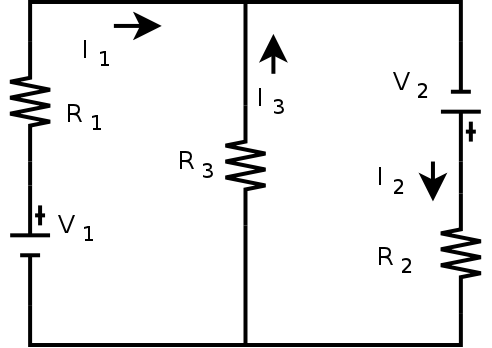It gives us the following three Kirchhoff's Law equations ( See the complete analysis here. ) :
-I1 R1 + I3 R3 = -V1

-I2 R2 -I3 R3 = -V2

-I1 + I2 -I3 = 0

A computer algebra system can be very useful for analyzing circuits like this.

8. ### Phasors and AC Circuits (external document)

1. Opening Maple:
• Open Maple from the menu or the desktop icon.

2. Solving Equations:
• To solve a set of Kirchhoff's Law equations, use the solve command, solving for I1, I2, and I3:

At the prompt, type in the the commands as shown: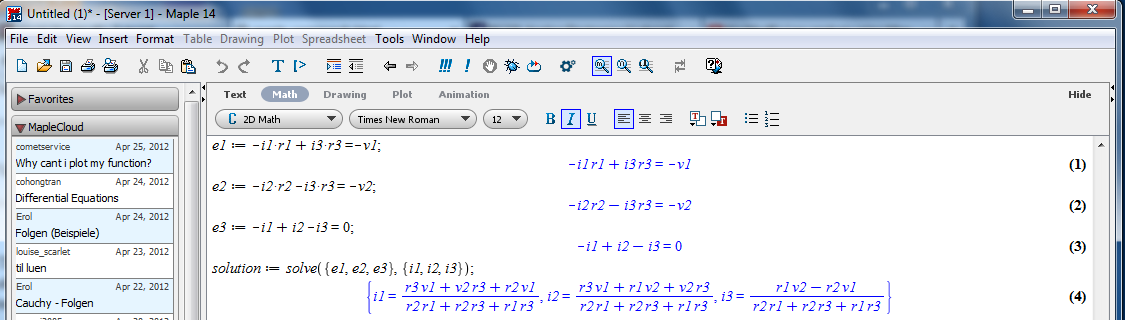The solve command gives the solution vector.

• The solution is a vector of currents. We can pick off a single current by using an index with the previous result: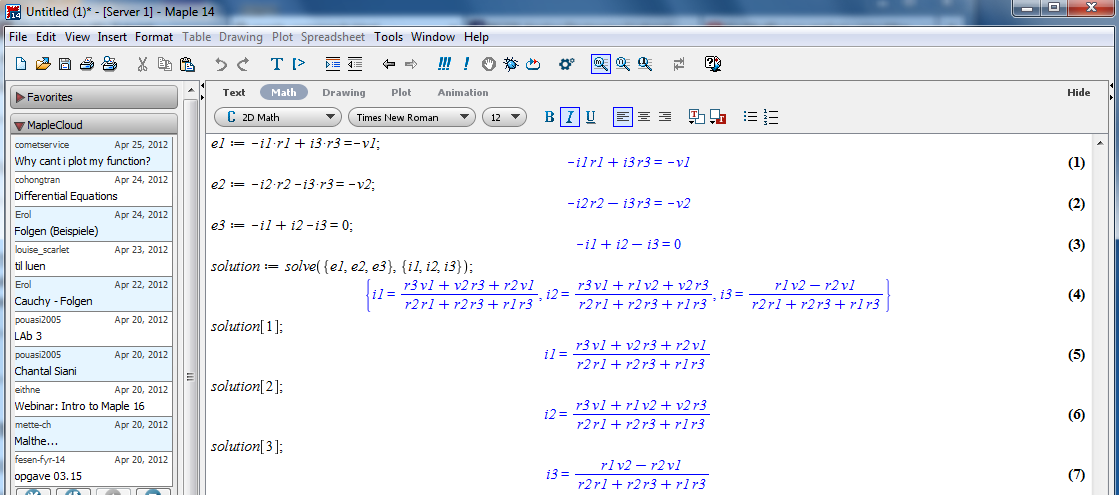• You can substitute in specific component values: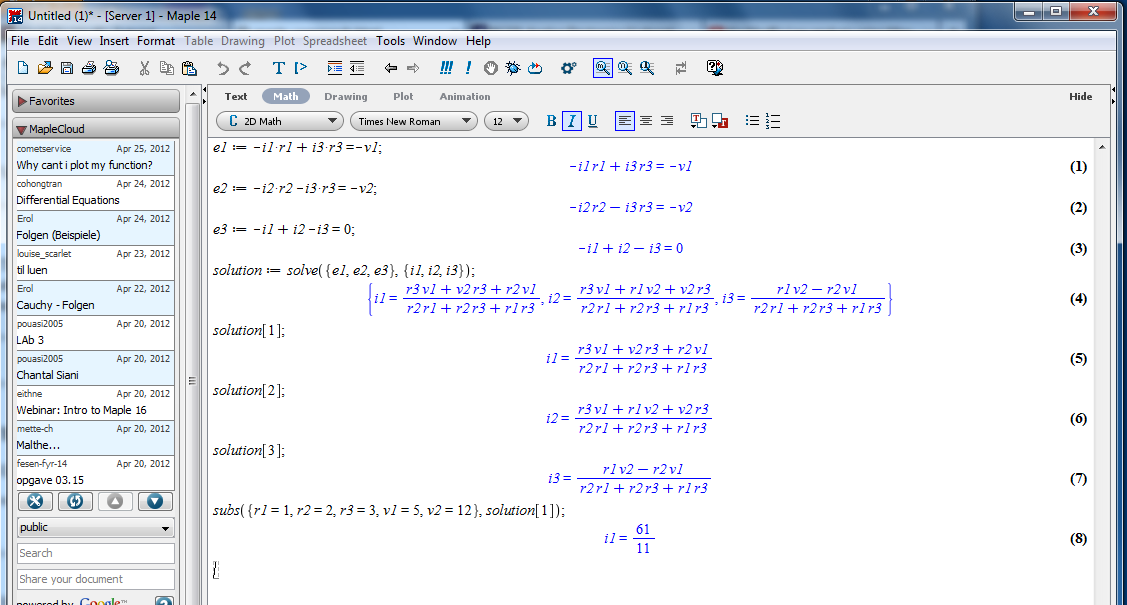If numbers are not integers, results will also be non-integer
Note that as soon as we use scientific notation, values become non-integer.

• We can get the voltage across a resistor by multiplying the current times the resistance, and assigning it to a new variable: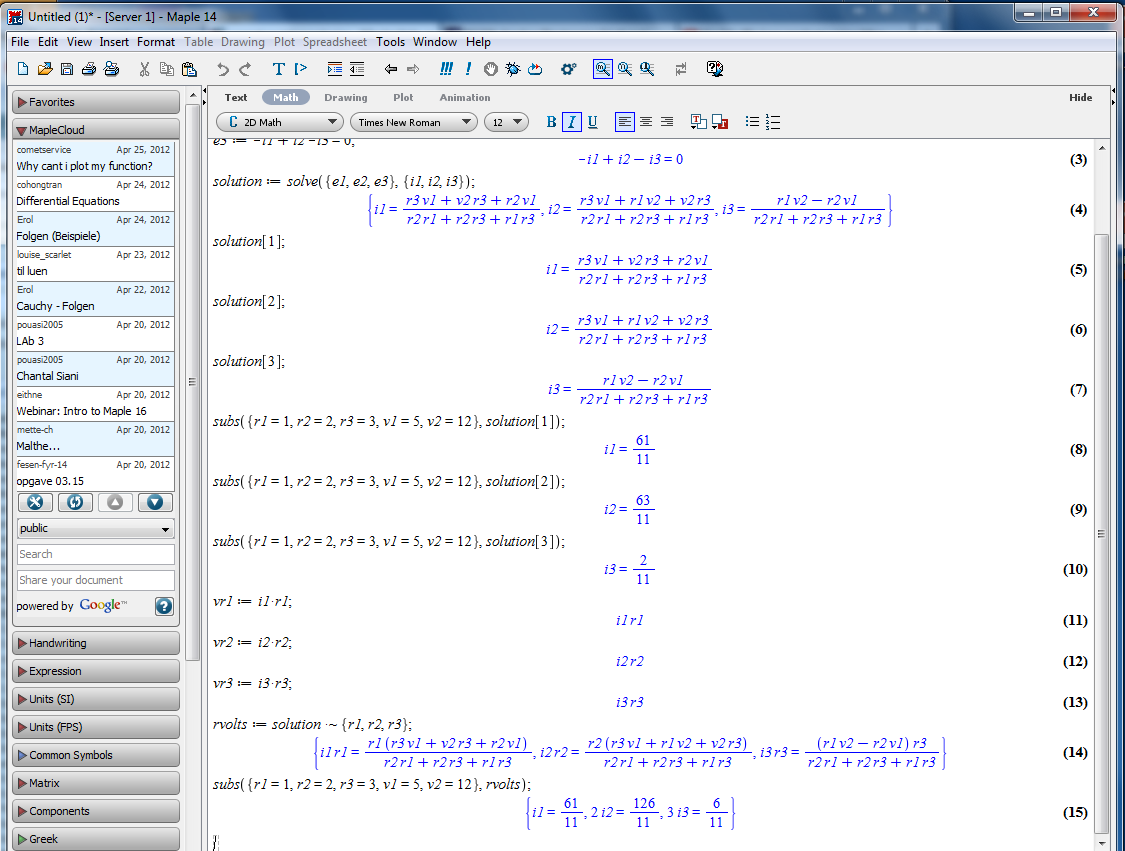Important tip: To combine two elements of two different arrays of the same size, just put a tilde after whatever operation you choose (such as +, -, etc.).

3. Saving a Session:
• This allows you to come back to it later with all of the variables the same.

To save a session: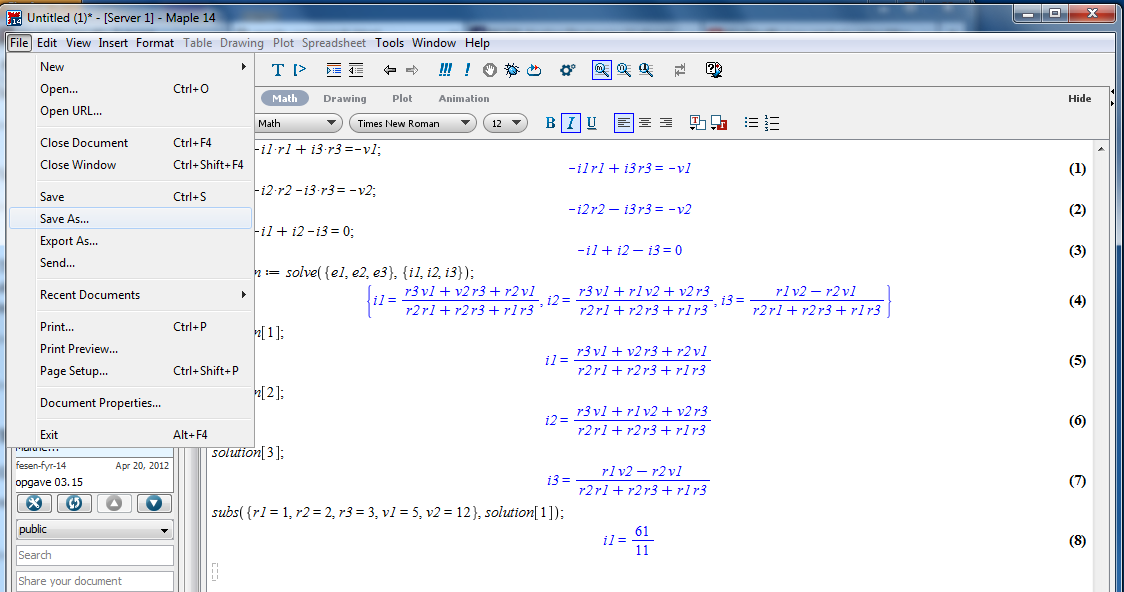4. Quitting Maxima:
• Quitting maxima:

• It's nice to be able to pick up where you left off, so you can keep developing an analysis over time.Everything should be as you left it.

• Previous statements can be re-executed and/or edited.

• Statements can be re-evaluated with different numbers.

6. Redefining Variables:
• Variables can be changed.

7. Getting Resistor Voltages:
• We can pick off individual current equations as well as just the results for each current:8. Phasors and AC Circuits (external document)## Resources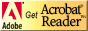If you need to update a browser, you might try Firefox which is
• free
• open source
• available for several platformsSince this page uses cascading style sheets for its layout, it will look best with a browser which supports the specifications as fully as possible.

If you are looking for an office package, with a word processor, spreadsheet, etc., you might try LibreOffice which is
• free
• open source
• available for several platforms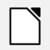Go to the main page for the Department of Physics and Computer Science.

Wilfrid Laurier University